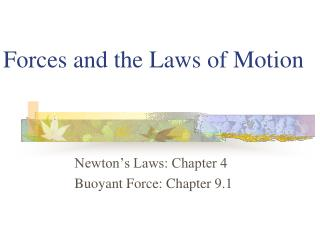# Forces and the Laws of Motion - PowerPoint PPT PresentationDownload PresentationForces and the Laws of Motion

Forces and the Laws of MotionDownload Presentation## Forces and the Laws of Motion

- - - - - - - - - - - - - - - - - - - - - - - - - - - E N D - - - - - - - - - - - - - - - - - - - - - - - - - - -
##### Presentation Transcript

1. Forces and the Laws of Motion Newton’s Laws: Chapter 4 Buoyant Force: Chapter 9.1

2. Quiz • Given: Force of 30N, east Force of 40N, north Find: resultant force: magnitude and direction

3. Changes in Motion • Force: a push or a pull • A push or pull changes the velocity of an object whether at rest or moving • Force increases directly as the mass of an object increases • Force increases directly as an object accelerates to a higher speed • In other words, Force = mass x acceleration

4. SI unit for Force • Force = mass (kg) x acceleration(m/s2) = kg m/s2 = Newton (N) Or Force = mass (g) x acceleration (cm/s2) = g cm/s2) = dyne 1lb. = 4.448N 1N = 0.225 lb.

5. Classes of Forces • Contact force: results from physical contact between two objects • Field force: does not require physical contact: ex: gravity, electrical charges

6. Force: a push or a pull

7. Force Diagrams

8. Try this one, part of homework 1. You are walking to class at a constant velocity. To move forward at 0 degrees you push back at 180 degrees with a force of 15N. If you weigh 600N (140lbs) draw a vector diagram of the forces acting on your body as you walk.

9. If Forces are balanced

10. Head to Tail Method

11. Parallelogram method

12. Try this one: also part of homework Given 3 vectors Vector A: 15N at 70 degrees Vector B: 20N at 150 degrees Vector C: 4kg at 270 degrees Diagram it and determine the resultant force by finding the horizontal and vertical components

13. Everyday Forces: Chapter 4-4 • Weight = Fg = mg = kg x 9.8m/s2 on earth • Normal force = Fnorm = Fn = opposite in direction to contact surface = mgcosΘ where Θ is the angle between the normal force and the vertical line.

14. Practice: Solve the following • Mr. Trotts is standing on a ramp that has a 15 degree slope to the ground. If Mr. Trotts weighs 100kg what is the normal force acting on him.

15. The Force of Friction • Static friction:(Fs) a force resisting objects at rest from moving by opposing forces applied attempting to set them in motion Fs = -Fapplied • Kinetic friction:(Fk) retarding frictional force on an object in motion • Net external force: force causing object to change motion = F – Fk

16. Coefficient of friction (µ) expresses the dependence of frictional forces on the particular surface they are in contact with • Coefficient of kinetic friction: µk = Fk/Fn • Fn = mgcosΘ • Coefficient of static friction: µs = Fsmax/Fn • Force of friction: Ff = µ Fn Try another one: part of homework Practice 4C page 145 #2 (use sample 4C)

17. Net Force • Forces up should equal forces down if no change in motion occurs up or down • 69.3N right minus 40N left = 29.3N right • If the object weighs 100N then its mass is weight/gravity = 100N/9.8m/s/s =about 10kg Acceleration = F/m = 29.3N/10kg = 2.93m/s/s to the right